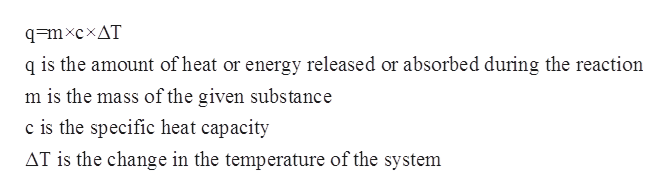# How much heat is released when a 260.0 g plate of copper tools from 5.30 x 10^2K to 20.0C?

Question
69 views
How much heat is released when a 260.0 g plate of copper tools from 5.30 x 10^2K to 20.0C?

check_circle

Step 1

The specific heat capacity for a given chemical substance or a solution refers to the amount of the heat that is required to increase the temperature of a given substance or system per unit of its mass. The mathematical relationship between specific heat capacity, mass, change in temperature and the heat of the solution is given by the following equation:help_outlineImage Transcriptioncloseq=mxcxAT q is the amount of heat or energy released or absorbed during the reaction m is the mass of the given substance c is the specific heat capacity AT is the change in the temperature of the system fullscreen
Step 2

The initial temperature is given to be 5.30×102K=530 K= 256.85 oC.

The final temperature is given to be 20oC.

The mass of Copper plate is given to be 260.0 g.

The specific heat capacity of...

### Want to see the full answer?

See Solution

#### Want to see this answer and more?

Solutions are written by subject experts who are available 24/7. Questions are typically answered within 1 hour.*

See Solution
*Response times may vary by subject and question.
Tagged in

### Physical Chemistry